## Consider the sequence shown. 436, 218, 109, 54.5, 27.25,… Select the correct explicit formula for the

Question

Consider the sequence shown.

436, 218, 109, 54.5, 27.25,…

Select the correct explicit formula for the sequence.

a
g_n=0.5·436^{(n-1)}

b
g_n=436·2^{(n-1)}

c
g_n=436·0.5^{(n-1)}

d
g_n=2·436^{(n-1)}

in progress 0
2 months 2021-07-27T02:17:27+00:00 1 Answers 4 views 0

1. Given:

The sequence is

436, 218, 109, 54.5, 27.25,…

To find:

The explicit formula for the given sequence.

Solution:

We have,

436, 218, 109, 54.5, 27.25,…

It is a GP because the two consecutive terms have common ratio.

Here,

First term : a=436

Common ratio : d =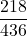=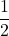=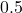Now, the explicit formula for a GP is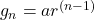Where, a is the first term and r is the common ratio.

Putting a=436 and r=0.5, we get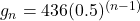Therefore, the correct option is c.